# Measurement of Reactance of a Shunt Reactor

The following two factors to be considered during measurement of reactance of a shunt reactor.

• The reactance of a shunt reactor is approximately equal to its impedance as the resistive component of the impedance in shunt reactor is negligible.
• The V-I characteristics of the shunt reactor is almost linear under operating range of applied voltage. This is because, gapped core is used in the shunt reactor to prevent magnetic saturation of the core within normal operational range.

The simple formula of impedance in ohm is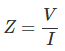Where, V is voltage in volt and I is current in ampere.

But in the case of shunt reactor, impedance Z = reactance X.
Hence, here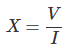Where, V is applied voltage across the winding of the reactor and I is the corresponding current through it.
As the V-I characteristic of the reactor is linear, reactance of the reactor winding remains fixed for any applied voltage below the maximum rated value.
In the case of reactance measurement of three phase shunt reactor, we use sinusoidal three phase supply voltage of power frequency (50 Hz) as test voltage. We connect three supply phases to three terminals of the reactor winding as shown. Before that we should make sure that the neutral terminal of the winding is properly earthed.

After switching on the supply, we measure the current flowing through each phase of the winding with the help of suitably sensitive clip on meter. After current measurements, we have to calculate the average current per phase. The average is taken as algebraic summation of three phase currents divided by 3. The measured reactance of the three phase shunt reactor is taken as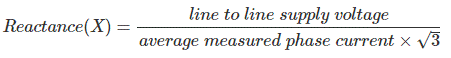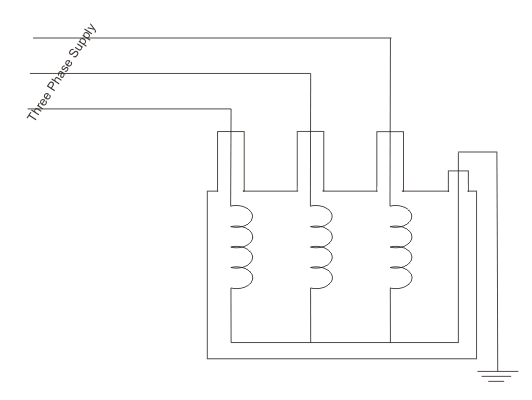For three phase reactors with magnetic iron path for zero sequence flux, zero sequence reactance may be measured as follows,
In that case the three terminals of the reactor are shorted and single phase supply is applied between common phase terminal and neutral terminal of the winding. After measuring the current through the common path we have to divide the applied single phase voltage by it. We then multiply 3 with the result to obtain zero sequence reactance per phase.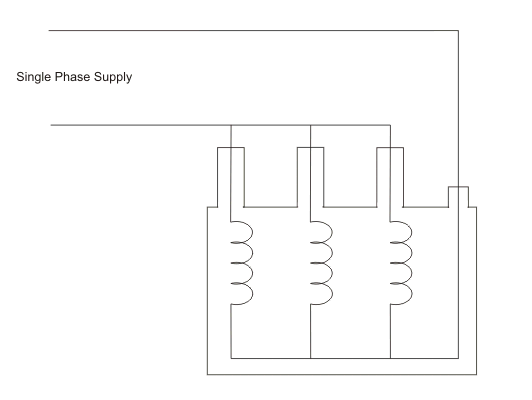Want To Learn Faster? 🎓
Get electrical articles delivered to your inbox every week.
No credit card required—it’s 100% free.